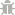Light Mode
Rate us!# speed converter

To convert velocity use our handy tool, select the velocity/speed unit and add the value you wish to transform to another unit of speed in the “From” field and select the unit in which you want to convert from the “To” field. The results will be displayed without any delay.

From: Foot/minute (ft/min)
To: Foot/minute (ft/min)

Have you ever wondered how you can convert speed measurements from one unit to another? Well, this doesn’t require you to memorize any formulae or manual calculations anymore. At Small SEO Tools, we have a speed converter, which is simply an online tool that converts the speed and velocity units such as m/sec, ft/min, sec, kilometer, Knot, Mile and Speed of light.

Speed Distance Time calculator made easy!

Obviously, there is an important speed distance time relation in the calculation of speed. Our speed conversion tool performs the function of a speed distance time calculator. It is easy to use and freely available online. You can simply convert any number of kilometers to Mph, Kph to Mph, Mph to Kmh, Kmh to Mph and Kilometers to Miles per hour into what should be its equivalent in miles per second.

## Why Should You Use a Speed Distance Calculator?

Speed has to do with how fast movement occurs, that is, distance in relation to time. One way of measuring the speed at which a moving object travels such as miles per hour (mph) might not be applicable. Because distance and time are important when we’re referring to motion and speed, we need to know the various units in talking about time, speed and distance.

Also, different countries have different units of measuring speed. In the US, the unit of speed is measured as miles per hour (mph), while the general SI unit is measured as kilometer per hour. One mile per hour is equal to 1.609344 kilometers per hour. Thus, you need a speed calculator because there are different units of distance; such as centimetres, feet, meters, miles, and so on. Also, because there are different units of time; such as seconds, minutes, and hours.

### Speed Calculator for Bike Speed and Wind Speed

You can calculate the speed for any moving object, not just for cars. You can use our online tool as a wind Speed Converter, a bike speed calculator or even human speed conversion utility. Wind speed varies widely in different geographic locations. Therefore, a wind speed conversion is needed where measurement has to be reported using different units, such as nautical miles per hour or statute miles per hour. Whatever your need, our speed distance calculator is just a click away from producing you the right answers.

### How To Use This Efficient Speed Converter to convert Kph to MPh, Mph to Kmh & Kmh to Mph

To use the tool, you have to follow only a few easy steps:
Step 1: First of all, visit https://smallseotools.com/speed-converter/

Step 2: Ensure you select the right tool of unit conversion. In this case, it indicates “Velocity & Speed Converter” at the top.

Step 3: Input the number of measurements you intend to calculate.

Step 4: Select the unit you’re converting from, (which comprises the distance/time e.g; kilometer/hour).

Step 5: Select the unit you want it converted into.

Step 6: The correct conversion value is automatically reflected in the box tagged “To”.

This process is very simple and is perfect to solve mathematical problems relating to speed. Not only that, it is a functional tool for amateurs and even professionals that require such conversions. Being able to convert the values of speed to different units quickly and correctly is thus made accessible on our website by following the steps given.

### AS SEEN ON:

Plagiarism Checker